# Power Query – Absolute and relative referencesIn Excel, we can easily create absolute and relative references.  A simple \$ symbol here and there is all it takes.  But Power Query is less flexible, therefore getting values from other rows is not as simple.  So, in this post, we cover how to get Power Query absolute and relative references.

I recommend you download the example file for this post.  Then you’ll be able to work along with examples and see the solution in action, plus the file will be useful for future reference.

## Absolute references

Look at the Absolute references tab in the example file.  In this worksheet, there are five scenarios to compare against a base.As shown in the screenshot above, the formula in cell C2 is:

`=B2-\$B\$2`

\$B\$2 is an absolute cell reference. When we copy the formula down, each cell consistently references cell B2.

Next, let’s see how we achieve a similar result in Power Query.  Having loaded the data, the Preview Window displays this:Power Query has already made some transformations for us.  There is no need to change any of these.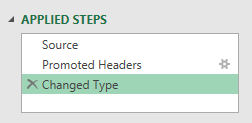Right-click on the first row in the Profit column, select drill down from the menu.Look at the formula bar (click View -> Formula Bar if it is not visible), it contains the syntax for referencing a specific row and column within Power Query.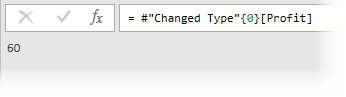The M code is:

`=#"Changed Type"{0}[Profit]`
• #”Change Type” is the name of the previous transformation step
• {0} references the first row of data.  In Power Query, counting always starts at zero.
• [Profit] is the name of the column.

This gives us the syntax to get the absolute reference for any item in the Power Query window.  Delete this step; we no longer need it.

Now we will add a custom column to replicate Excel’s calculation (as shown above).  Click Add Column -> Custom Column.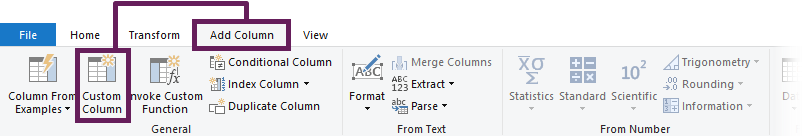In the Custom Column dialog box, enter the following formula.

`=[Profit] - #"Changed Type"{0}[Profit]`

Enter a new column name, such as Vs Base (PQ), then click OK.That’s it.  Look at the screenshot below.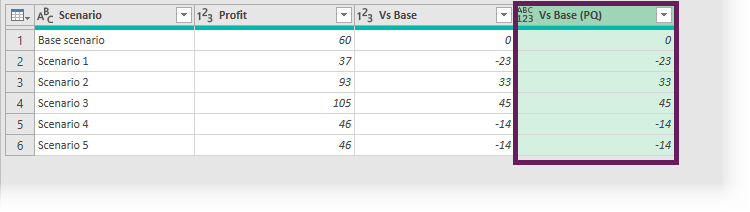We now have a formula that is always referencing a specific row of a specific column.  In the screenshot above, the Vs Base (PQ) is calculating the same value as we calculated in Excel in the Vs Base column.

100 Excel VBA Macros

Do you know the fastest way to learn foreign languages?  It is to read, write, speak, and think in that language as often as possible.  Apart from speaking, programming languages are no different.  The more you immerse yourself in that language, the faster you will pick it up.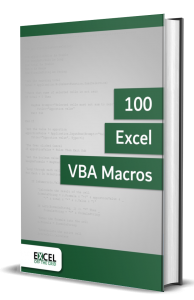Therefore, what most people like you need is lots of examples that you can practice.  That is why the 100 Excel VBA Macros eBook exists.  It’s the book for all Excel users who want to learn how to read and write Excel macros, save time, and stand out from their peers.  The book contains:

• 100 example codes to practice reading and writing macros that will embed the language into your thinking.
• An introduction to macros in Excel to ensure you can implement the VBA code in the book even if you have no prior knowledge.
• Consistent code layout between examples to enable you to understand the structure and easily customize the code to meet your needs.
• Downloadable workbook containing all the source code, so the examples can be added to your project to give you the benefit of VBA straight away.

## Relative References

Look at the Relative references tab in the example file.  In this example, we have five years of profits and a base year.  The goal is to create a column showing the increase in Profit since the prior year.

The prior year is a relative reference, as the cell changes for each formula (i.e., in our example, the prior year is always one row above).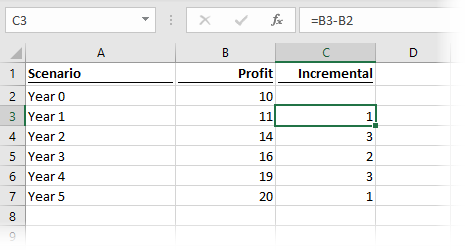As shown in the screenshot above, the formula in cell C3 is:

`=B3-B2`

As the formula is copied down, the cells referenced in the formula change at a relative rate.  As a result, when copied down one cell B3 becomes C3, then when copied down again it becomes D3.

Now it’s Power Query’s turn to create a relative reference.  After loading the data into Power Query, the Preview Window looks like this: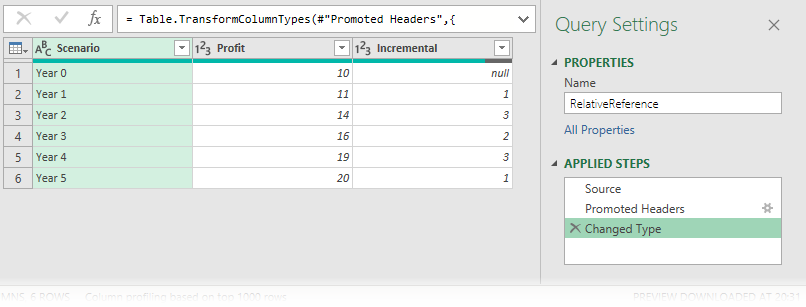Add an Index column by clicking Add column -> Index Column (drop-down) -> From 0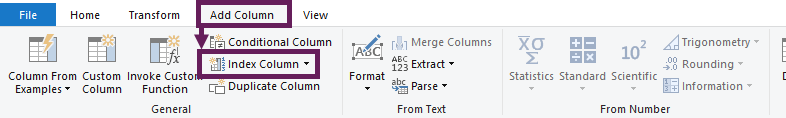In the dialog box, enter this formula.

`=[Profit] - #"Added Index"{[Index]-1}[Profit]`

The Index column is used to determine which row is used in the formula.

Give the column a name such as Incremental (PQ), then click OK.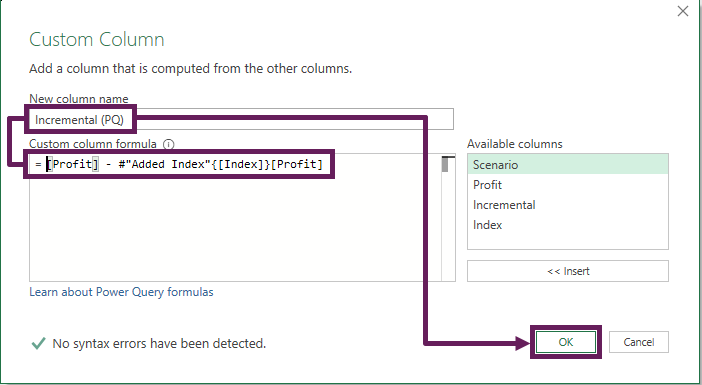The Preview Window looks like this: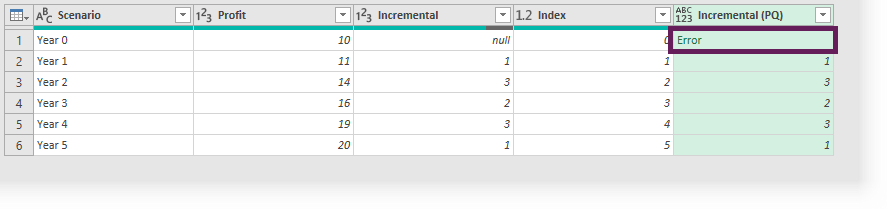As we are referring to the row above, the first row can never have a value; as a result, Power Query displays an error.

Select the new Incremental (PQ) column, then from the ribbon click Transform -> Replace Values (drop-down) -> Replace Errors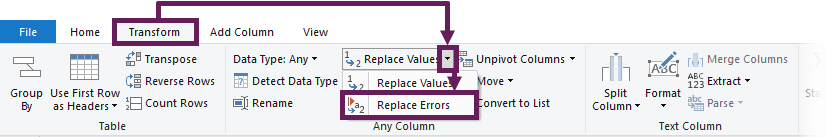In the Replace Errors window, enter null in the Value box, then click OK.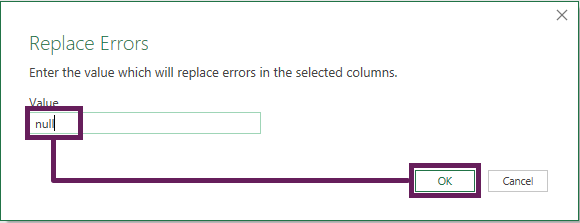And we’re done.  It wasn’t too hard, after all.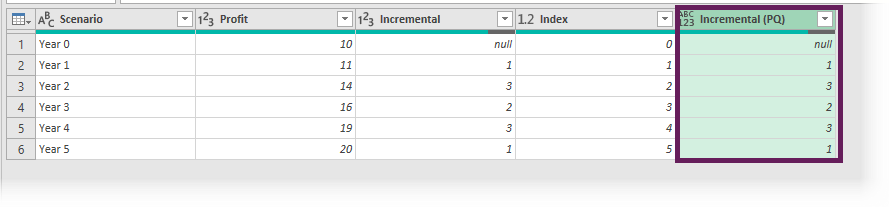In the screenshot above, the Incremental (PQ) column calculated the same value as we had in Excel (which is the Incremental column).  Therefore, we have created a relative reference 🙂

## Conclusion

Power Query is not as flexible as standard Excel.  This is a strength because it means the data structure is more robust.  But, to replicate some simple Excel formulas, we need to work a bit harder.  As we’ve seen, while absolute and relative references are not standard Power Query transformations, we can write a simple formula to achieve the same outcome.Get our FREE VBA eBook of the 30 most useful Excel VBA macros.
Automate Excel so that you can save time and stop doing the jobs a trained monkey could do.

Email Address * First Name *

Don’t forget: